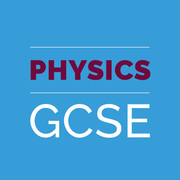# Learn Force And Motion

Study Force And Motion using smart web & mobile flashcards created by top students, teachers, and professors. Prep for a quiz or learn for fun!

### Top Force And Motion Flashcards Ranked by Quality

• Physics 1 - Motion, Forces And Conservation Of EnergyShow Class
• Motion and ForcesShow Class
• Physics - Forces and MotionShow Class
• physics forces and motion Y7 and 8Show Class
• Forces & Motions 101Show Class
• Forces and MotionShow Class
• forces and motionShow Class
• Science - Forces and MotionShow Class
• Physics: Forces and MotionShow Class
• PHYSICS- Forces And motionShow Class
• forces and motionsShow Class
• P10. Forces and MotionShow Class
• Forces and MotionShow Class
• Physics A - Forces and MotionShow Class
• Motion And ForcesShow Class
• Motion, Forces, and Newton's lawsShow Class
• A-level Physics- forces and motionShow Class
• Force & MotionShow Class
• Motion And ForcesShow Class
• Motion And ForcesShow Class
• Forces, Motion, and EnergyShow Class
• Science/Forces & MotionShow Class
• Motion And ForcesShow Class
• Motion And ForcesShow Class
• Motion and ForcesShow Class
• Motion And ForcesShow Class
• Motion and forcesShow Class
• Motion And ForcesShow Class
• Motion and forcesShow Class
• Unit 2: Motion and ForcesShow Class
• Motion and ForcesShow Class
• Physics - Forces and MotionShow Class
• Motion and forcesShow Class
• Spencer Chapter 6: Forces and MotionsShow Class
• 5th grade Science Force and Motion and WorkShow Class
• Motion and forcesShow Class
• Physics Paper 2: Chapter 10 - Force And MotionShow Class
• PhysicsShow Class
• Bixel AP PhysicsShow Class
• Edexcel GCSE PhysicsShow Class
• PhysicsShow Class
• Edexcel GCSE Physics (9-1)Show Class
• ScienceShow Class
• GCSE physicsShow Class
• OCR A-Level PhysicsShow Class
• iGCSE PhysicsShow Class
• Year 11 Science - Mechanics (AS90940)Show Class
• Year 9 ScienceShow Class
• Year 9 Science 2020Show Class
• RGS 3rd Form Physics 4PH-DShow Class